### Multidimensional differential equations and field conversions

At first let's see some formulaes of differential operators in vector algebra:

• grad (gradient) gives us the direction of fastest increase of function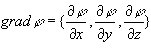• div (divergence) is a thread of vector from very little area relative to it's space (for example, a thread of vector of velocity of liquid has very simple meaning)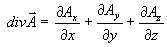• rot (rotor) is a circulation of vector on a very small contour relative to it's square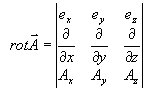Here is some expressions, reflecting the relations between this operators:
• div(fA) = f*div(A) + A*grad(f)
• rot(fA) = f*rot(A) - [grad(f),A]
• div([A,B]) = B*rot(A) - A*rot(B)
• rot(grad(f)) = 0
• div(rot(A)) = 0

#### Laplas and D'Alamber equations

The most important equation is Laplas equation (or Puasson equation as more generalised type of equations). Laplas and Puasson equations is equations for such fields:

• field of electric potential
• field of temperature
• field of pressure
• field of "velocity potential" in hydrodynamics
• and many others, where div(grad(f))=g(x,y,z)
Laplas Equation is: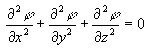Puasson equation is: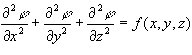D'Alamber equation is: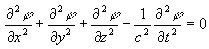When the field is approximated by the matrix (for example, Aij), Laplas or Puasson equations takes such form:
(Aij - Aij-1) + (Aij - Aij+1) + (Aij - Ai-1j) + (Aij - Ai+1j) = Cij
where Cij is the matrix of density (for Laplas equation, Cij =0). So you can simply solve this equations by setting boundary conditionds on the first step and by obtaining the next value for [i,j] element as:
Aij = (Cij + Aij-1 + Aij+1 + Ai-1j + Ai+1j)/4
The matrix will match better and better to the equation on each next step.

D' Alamber equation is an equation for running waves and is very similar to Laplas equation, and you can solve it both in complex Minkouski space and by approximating continual space by discrete dynamic elements, moving according to Newton's laws.

Here is a sample Delphi project, solving Laplas equation Laplas.pas and sample Pascal program emulating waves on liquid surface Waves.pas.

#### From matrixes and vectors to multidimensional functions

We can simply generalise all methods of matrix algebra onto functions.
So scalar multiplication of functions is: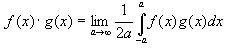"Multiplication of vector by matrix":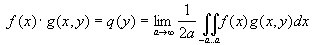Basis function is ortogonal if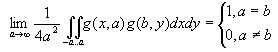As you see now, Fourier analysis is only translating function as "vector" to another basis. Now Fourier method is almost evident: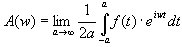You can check whether the "basis function" f(w,t) = eiwt is ortogonal.

#### Random oscillations

Random oscillations which can be obtained from Random function in Delphi is so-called "white noise" with proportionally distributed spectrum. The most common models for random oscillations with non-proportional spectrum is Lorens model, which reflects the behaviour of heated liquid in circle tube with counting of gravitational field. It is given by such a system of differential equations: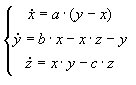I used parameters a=10 b=30 c=2.667. One more way to model random oscillation is modelling radiowave generator with non-linear element such as "tonnel diode" and others. It is given by such a system of equations (here Van-der-Pohl model for generator is accepted):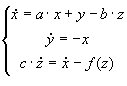where f(x) is a dependence between voltage and current in the diode.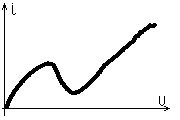This dependance must have such a "pit" to produce random oscillations.

Here is the sample code emulating random oscillations according to Lorenz model Random.pas

#### Fourier analysis

Fourier analysis is performed by such a translation (see p. 2). To do this on the computer, here is a formulae: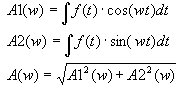It can be used, for example, to compress sound files.

Here is the sample code performing Fourier analysis Fourier.pas

#### Sound processing

A simple sound processing program was written to demonstrate all it. It's a demo program, so you are able to work only with mono ".wav" files, and you should not also use big characters in Formulae Generator and small characters in Rhythm Composer. Such functions is available in Formulae Generator (Generator 1 on the toolbar):

• sin(t),cos(t) - harmonic functions
• rcn(t) - rectangular wave with period of 1
• ran(t) - triangular wave with period of 1
• sqr(t) - square root (if t<0,sqr(t)=0)
• rand(x) - random with level x
• amp(t) - amplitude at time t
• exp(t), ln(t) - logarithmic and exponential functions
Unit Wave performs basic writing and reading wave files functions, unit Calculator is a formulae compiler, some additional functions like Echo, Filters, Noise and others can be found in unit Generator.

Here is the sample Delphi project on sound processing Sound.zip

©2002-2003, Veter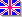English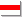Беларуская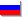Русский
Сайт создан в системе uCoz